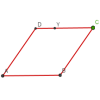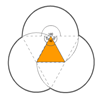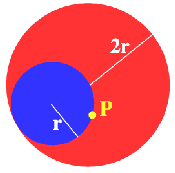You may also likeRoaming Rhombus

We have four rods of equal lengths hinged at their endpoints to form a rhombus ABCD. Keeping AB fixed we allow CD to take all possible positions in the plane. What is the locus (or path) of the point D?Rolling Coins

A blue coin rolls round two yellow coins which touch. The coins are the same size. How many revolutions does the blue coin make when it rolls all the way round the yellow coins? Investigate for a line of 'n' coinsTriangles and Petals

An equilateral triangle rotates around regular polygons and produces an outline like a flower. What are the perimeters of the different flowers?

Just Rolling Round

Age 14 to 16 Challenge Level:Why do this problem? In this problem , the interactivity enables learners to experiment and make their own conjectures about the locus. The proof requires only simple geometrical reasoning involving circle theorems and arc lengths. Possible approach Learners might first experiment with the interactivity and make a conjecture about the locus, then try to prove their conjecture.

Key question
We know the small circle rolls around inside the big one. What can we say about arc lengths?

Possible support
Learners might draw their own interactive diagrams using Geogebra.

Ask about how far a bicycle goes forward when the wheels rotate through exactly one revolution.

What about a revolution by a given angle?

What if the road was curved?

Possible extension
See the problem Illusion.